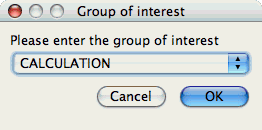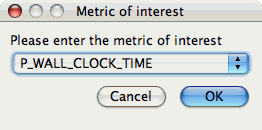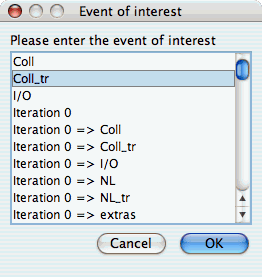## 22.1. Setting Parameters

There are a few parameters which need to be set when doing comparisons between trials in the database. If any necessary setting is not configured before requesting a chart, you will be prompted to set the value. The following settings may be necessary for the various charts available:

### 22.1.1. Group of Interest

TAU events are often associated with common groups, such as "MPI", "TRANSPOSE", etc. This value is used for showing what fraction of runtime that this group of events contributed to the total runtime.

Figure 22.1. Setting Group of Interest### 22.1.2. Metric of Interest

Profiles may contain many metrics gathered for a single trial. This selects which of the available metrics the user is interested in.

Figure 22.2. Setting Metric of Interest### 22.1.3. Event of Interest

Some charts examine events in isolation. This setting configures which event to examine.

Figure 22.3. Setting Event of Interest### 22.1.4. Total Number of Timesteps

One chart, the "Timesteps per second" chart, will calculate the number of timesteps completed per second. This setting configures that value.

Figure 22.4. Setting Timesteps